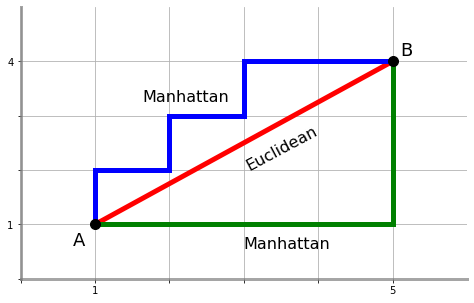# Manhattan Distance Calculator

Created by Rijk de Wet
Reviewed by Hanna Pamuła, PhD candidate and Jack Bowater
Last updated: Nov 26, 2021

Are you wondering how far you have to walk to school? Maybe you're planning the route for your morning jog? Or are you just sick and tired of plain old Euclidean geometry?

Welcome to the Manhattan distance calculator. Here, you will learn what the Manhattan distance is, what it is used for, and how to calculate the Manhattan distance between two coordinates of your own.

## What is the Manhattan distance?

The Manhattan distance is a distance metric between two points. It's the sum of the absolute differences between these points' coordinates. It's also known by other names:

• The taxicab distance;
• The city block distance; and
• The snake distance.

Picture this: you're in a city like New York or San Francisco, where the streets are neatly laid out in a grid. To get from point A to point B, you have to walk from intersection to intersection along the grid — you can't walk diagonally through the city's blocks. In mathematical terms, you're constrained to traveling along the city's coordinate axes, unlike a bird who can just fly straight there. The number of city blocks you'd have to walk is called the Manhattan distance, and you can probably already see where its other names — taxicab distance, city block distance, and snake distance — come from.The Manhattan distance and the Euclidean distance between points A(1,1)A(1,1)A(1,1) and B(5,4)B(5,4)B(5,4). The Manhattan distance is longer, and you can find it with more than one path.

The Pythagorean theorem states that $c = \sqrt{a^2+b^2}$. While this is true, it gives you the Euclidean distance. If you were to rewrite the Pythagorean theorem for the Manhattan distance, it would instead be $c = a + b$.

## Why is the Manhattan distance used?

Beyond determining how far away that coffee shop is in New York City, the Manhattan distance has a surprising number of applications. Here are just a few:

• In chess, the distance between squares for a rook is measured with the Manhattan distance, as it can only travel horizontally and vertically.

• In data science and machine learning, the Manhattan distance is used in speech recognition and image processing.

• In molecular biology, the Manhattan distance is used to decide where to splice genes and other molecules.

• In the popular Snake video game genre, the number of steps your snake has to move towards the next food item can be determined with the Manhattan distance; this is how it earned the nickname "snake distance".

## What is the formula for the Manhattan distance?

With two N-dimensional points $\vec{a} = \left[ a_1, \dots, a_N \right]$ and $\vec{b} = \left[ b_1, \dots, b_N \right]$, the Manhattan distance $d$ can be calculated as:

$d = \vert a_1-b_1\vert + \dots + \vert a_N - b_N\vert$

Let's see how to calculate the Manhattan distance for a real-world example set in real-world Manhattan. Manhattan's east-west roads are called "streets", and its north-south roads are called "avenues". Let's say you live on the corner of 9th Street and 2nd Avenue, thus making your starting point $\vec{a} = [2, 9]$. You want to buy some milk at the grocery store on the corner of 5th Street and 3rd Avenue, so your destination is $\vec{b} = [3, 5]$. Using the Manhattan distance formula, the total distance you'd have to walk along the streets of New York is

$\begin{split} d &= \vert a_1 - b_1 \vert + \vert a_2 - b_2 \vert \\ &= \vert 2-3 \vert + \vert 9-5 \vert \\ &= 1 + 4 = 5 \end{split}$

city block lengths.

It might become clear now that it doesn't matter exactly how you walk from point A to point B. You can walk down to 5th Street first (covering the four blocks) and then go to 3rd Avenue (covering the last block), or you can go to 3rd Avenue before going to 5th Street. In the end, you're going to walk five city blocks — see why it's called the "city block distance"?

## How to use the Manhattan distance calculator

The Manhattan distance calculator is easy to use. It first asks you how many dimensions your points have, allowing points in up to four-dimensional space. You can then simply enter your points into the calculator, and it will output the Manhattan distance between your two points.

## FAQ

### What is the difference between Manhattan distance and Euclidean distance?

The difference between these distance measures is the axial constraints. With Euclidean distance, the distance between point A and point B is the length of a straight line drawn between these points. Manhattan distance instead seeks the shortest path that is parallel to the coordinate axes system, and that path may end up not being straight.

### What is the relationship between Manhattan distance and Euclidean distance?

As the Manhattan distance is constrained to move along the axes, it has to move further than the Euclidean distance does. The Manhattan distance can therefore never be shorter than the Euclidean distance between point A and point B.

Rijk de Wet
Dimensionality
2D
First Point
x
y
Second point
x
y
Distance
Distance
People also viewed…

### Circumference

Use this free circumference calculator to find the area, circumference and diameter of a circle.

### Eggs

Quantum physicist's take on boiling a perfect egg.

### Reciprocal

This reciprocal calculator is able to determine the reciprocal of any number (except 0, of course).

### Rectangle diagonal angle

The rectangle diagonal angle calculator helps you find the angles created by the diagonals of a given rectangle.